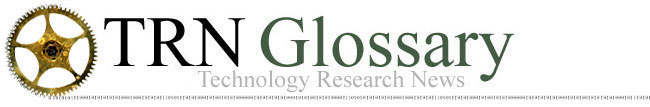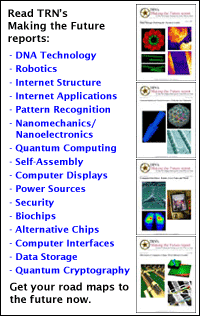A   B   C   D   E   F   G   H   I   J   K   L   M   N   O   P   Q   R   S   T   U   V   W   X   Y   Z

Quantum Computing: The experimental field of quantum physics and computer science that manipulates the quantum states of subatomic particles, atoms and molecules to perform the basic logic operations of computing. Because particles spin in one of two directions -- up or down -- and these can represent the ones and zeros of binary computing, particles can be used as quantum bits or "qubits." In its quantum state, a particle is not spinning in one direction but has a probability of spinning in either direction when it leaves the quantum state. This means that a qubit represents both zero and one at the same time. Two qubits represent every possible two-bit number (00, 01, 10, 11). Each additional qubit increases the number of possible numbers exponentially: n qubits represent 2n possible numbers. Twenty qubits represent every number from 0 to more than one million, 30 qubits represent every number from 0 to more than one billion, and 40 qubits represent every number from 0 to more than one trillion. A quantum computer acts on a set of particles by influencing the probabilities of the particles' spins (posing the problem) so that when the particles leave their quantum state the resulting spins represent a specific number (getting the answer). Quantum computers have the potential to be much faster than today's supercomputers because they operate on all the particles -- and thus all the possible numbers -- simultaneously. So far, researchers have been able to make quantum computers of only seven qubits, but that has been enough for them to confirm the basic principles of quantum computing.

Qubit: Quantum Bit. Qubits are individual atoms or subatomic particles. They are the basic units of quantum computing. Particles spin in one of two directions, which can represent the ones and zeros of binary computing.

A   B   C   D   E   F   G   H   I   J   K   L   M   N   O   P   Q   R   S   T   U   V   W   X   Y   Z